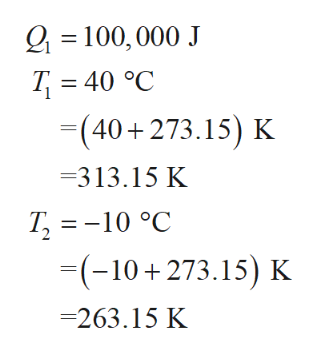# A heat engine operating between -10 degrees Celsius and 40 degrees Celsius achieves 50% of the ideal Carnot efficiency. If it takes in 100,000 J of heat in one heat cycleA. How much heat is exhausted in one cycle?B. How much work is done in one cycle?C. What was the entropy change of the high temperature reservoir? The low temperature reservoir?D. Was this cycle a reversible process?

Question
116 views

A heat engine operating between -10 degrees Celsius and 40 degrees Celsius achieves 50% of the ideal Carnot efficiency. If it takes in 100,000 J of heat in one heat cycle

A. How much heat is exhausted in one cycle?

B. How much work is done in one cycle?

C. What was the entropy change of the high temperature reservoir? The low temperature reservoir?

check_circle

star
star
star
star
star
1 Rating
Step 1

Hello. Since your question has multiple sub-parts, we will solve first three sub-parts for you. If you want remaining sub-parts to be solved, then please resubmit the whole question and specify those sub-parts you want us to solve.

Let T1 and T2 be the temperature of the source and the sink respectively and let Q1 amount of heat is taken from the source.help_outlineImage TranscriptioncloseQ100, 000 J T 40 °C =(40+273.15) K =313.15 K T, -10 °C -(-10 273.15) K -263.15 K fullscreen
Step 2

write the ideal engine’s efficiency(η’) formula and plug the suitable values to determine the ideal engine’s efficiency.

Step 3

Since, it is given that the efficiency of heat engine is 50% of the ideal engine’s ef...

### Want to see the full answer?

See Solution

#### Want to see this answer and more?

Solutions are written by subject experts who are available 24/7. Questions are typically answered within 1 hour.*

See Solution
*Response times may vary by subject and question.
Tagged in

### Thermodynamics